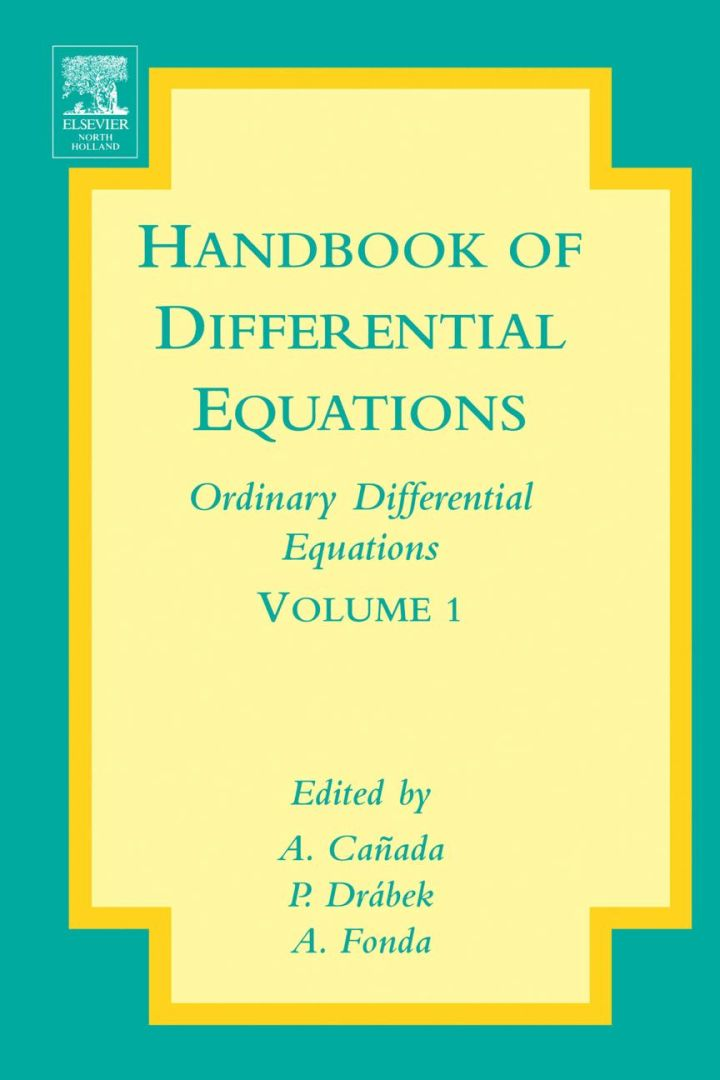# Handbook of Differential Equations: Ordinary Differential Equations

## Handbook of Differential Equations: Ordinary Differential Equations

By Canada, A.; Drabek, P.; Fonda, A.

### Rent or Buy eTextbook

Expires on Apr 13th, 2022
\$270

Publisher List Price: \$279.99
Savings: \$9.99

The book contains seven survey papers about ordinary differential equations.

The common feature of all papers consists in the fact that nonlinear equations are focused on. This reflects the situation in modern mathematical modelling - nonlinear mathematical models are more realistic and describe the real world problems more accurately. The implications are that new methods and approaches have to be looked for, developed and adopted in order to understand and solve nonlinear ordinary differential equations.

The purpose of this volume is to inform the mathematical community and also other scientists interested in and using the mathematical apparatus of ordinary differential equations, about some of these methods and possible applications.

Subject: Mathematics & Statistics -> Mathematics -> Mathematics GeneralPublisher: Elsevier S & T 09/2004
Imprint: North Holland
Language: English
Length: 708 pages

ISBN 10: 0444511288
ISBN 13: 9780444511287
Print ISBN: 9780444511287

Live Chats